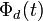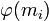# Order of periodic element of general linear group over integers is bounded

This article gives the statement, and possibly proof, of a particular group or type of group (namely, General linear group over integers (?)) satisfying a particular group property (namely, Group in which all elements of finite order have a common bound on order (?)).

## Statement

Suppose$n$ is a natural number. Then, the order of any element of the General linear group (?)$GL(n,\mathbb{Z})$ having finite order is bounded by a function of$n$.

Explicitly, if there exists an element of order$m$, then we can write$n = a_1\varphi(m_1) + a_2\varphi(m_2) + \dots + a_r\varphi(m_r)$ where$\varphi$ denotes the Euler totient function, all$a_i \ge 1$, and the lcm of$m_1,m_2,\dots,m_r$ equals$n$.

This gives a bounding function of the form: the maximum possible order of a$n \times n$ matrix is at most$(1 + n)^{2n}$. In practice, the maximum possible order is much lower.

## Particular cases

Value of$n$ Possible orders of periodic elements in$GL(n,\mathbb{Z})$ Maximum possible order lcm of possible orders
1 1,2 2 2
2 1,2,3,4,6 6 12
3 1,2,3,4,6 6 12
4 1,2,3,4,5,6,8,10,12 12 120
5 1,2,3,4,5,6,8,10,12 12 120
6 1,2,3,4,5,6,7,8,9,10,12,14,15,20,24,30 30 2520

## Proof

### Proof of the Euler totient function partition condition

Given: An element$g \in GL(n,\mathbb{Z})$ of order$m$.

To prove: There exists a partition$n = a_1\varphi(m_1) + a_2\varphi(m_2) + \dots + a_r\varphi(m_r)$ where the lcm of$m_1,m_2,\dots,m_r$ is$m$ and$\varphi$ is the Euler totient function.

Proof:

Step no. Assertion/construction Facts used Given data used Previous steps used Explanation
1$g$ satisfies the polynomial$t^m - 1$, so the minimal polynomial of$g$ divides$t^m - 1$.$g$ has order$m$
2 The minimal polynomial of$g$ is a product of cyclotomic polynomials$\Phi_{m_1}(t)\Phi_{m_2}(t)\dots \Phi_{m_r}(t)$, where the lcm of$m_1,m_2,\dots,m_r$ is$m$.$t^m - 1 = \prod_{d \mid m} \Phi_d(t)$,$\Phi_d(t)$ are irreducible$g$ has order$m$. Step (1) By Step (1) and the factoring of$t^m - 1$ we conclude that the minimal polynomial must be a product of$\Phi_d(t)$ for a subset of the set of divisors of$m$. Call these divisors$m_1,m_2,\dots,m_r$. They all divide$m$. If the lcm is smaller than$m$, then the order of$g$ is strictly smaller than$m$, a contradiction. Hence the lcm must be exactly$m$.
3 The characteristic polynomial is of the form$\Phi_{m_1}(t)^{a_1}\Phi_{m_2}(t)^{a_2}\dots \Phi_{m_r}(t)^{a_r}$ where$a_1,a_2,\dots,a_r$ are all positive integers, and the lcm of$m_1,m_2,\dots,m_r$ is$m$. minimal polynomial divides characteristic polynomial Step (2) Follows from fact and Step (2).
4$n = a_1\varphi(m_1) + a_2\varphi(m_2) + \dots + a_r\varphi(m_r)$, where$a_1,a_2,\dots,a_r$ are all positive integers, and the lcm of$m_1,m_2,\dots,m_r$ is$m$. degree of cyclotomic polynomial$\Phi_d$ is$\varphi(d)$, degree of characteristic polynomial of$n \times n$ matrix is$n$ Step (3) Direct from Step (3), compute degree of characteristic polynomial in two different ways.

### Proof of bounding

Given:$n = a_1\varphi(m_1) + a_2\varphi(m_2) + \dots + a_r\varphi(m_r)$, where$a_1,a_2,\dots,a_r$ are all positive integers, and the lcm of$m_1,m_2,\dots,m_r$ is$m$.

To prove:$m \le n^{2n}$.

Step no. Assertion/construction Facts used Given data used Previous steps used Explanation
1 Each$\varphi(m_i)$ is bounded by$n$.
2 Each$m_i.$x \le (1 + \varphi(x))^2$ for any natural number$x$. Step (1)
3$r \le n$
4$m$, defined as the lcm of$m_1,m_2,\dots,m_r$ is at most$m_1m_2\dots m_r$.
5$m \le (1 + n)^{2n}$ Steps (3), (4), (5) Step-combination direct.